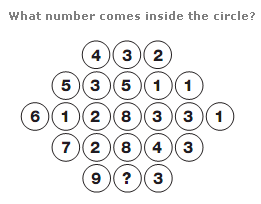## Gold box problem

There are ‘n’ gold boxes placed in a row, each having different number of gold coins.
2 players play a game, where the motive is to collect the maximum number of gold coins. Each player can see how many coins are present in each box, but can get a box from either end only, on his turn.
Design a strategy such that Player1 wins (Assuming both players play smartly)

Source: Amazon Interview

Find Solution here

## Duck and Fox

A duck is swimming at the center of a circle-shaped lake. A fox is waiting at the shore, not able to swim, willing to eat the duck. It may move around the whole lake with a speed four times faster than the duck can swim. Can the duck always reach the shore without being eaten by the fox?

Source: Raphael Reischuk Riddles

## 2 Goats and 1 Car

You are on a game show and there are three doors. The presenter tells you that behind one of doors there is a car and behind the other two are goats. If you pick the car you win it. After you have picked a door the presenter opens a different door with a goat behind it, he then gives you the chance to change what door you open. What should you do?

Hint: It is not 1/2 as you would first think.

## One Cord

You are given one cord that burns exactly one hour, not necessarily with constant speed.
How should you light the cord in order to determine a time interval of 15 minutes?
(Hint: solve the Two Cord puzzle first.)

Source: Raphael Reischuk

## Number of ordered Pairs

Let S be a set of n consecutive natural numbers.
How to find the number of ordered pairs (A,B), where A and B are subsets of S and A is a proper subset of B?

For Example:
Let the set S = {1, 2, 3, 4, 5, 6, 7, 8}.
Find the number of ordered pairs (A, B), where A and B are subsets of S and A is a proper subset of B.

Source: www.testfunda.com

Find Solution here

## Predict the other’s coin

Assume the following 3-player game consisting of several rounds. Players A and B build a team, they have one fair coin each, and may initially talk to each other. Before starting the first round, however, no more communication between them is allowed until the end of the game. (Imagine they are separated in different places without any communication infrastructure.) A round of the game consists of the following steps:

(1) the team gives one dollar to player C.
(2) Both A and B toss their coins independently.
(3) Both A and B try to predict the other’s coin by telling the guess to C. (No communication: A does not know the outcome of B’s coin toss, and vice versa, nor the guess).
(4) If C verifies that both A and B guess the other’s coin correctly, then C has to give 3 dollars back to the team.
Should C play this game?

Source: Raphael M. Reischuk

Find Solution here

## Number of paths

For a rectangular grid having a x b cells as shown in the below figure:-

Find the number of shortest paths along the edge of the cells. Starting from the bottom-left point and end at right-up point.
For simplicity they are marked as (0,0) and (a,b)
Write a pseudo code to print all such
paths.

Find Solution here

## Number inside Circle